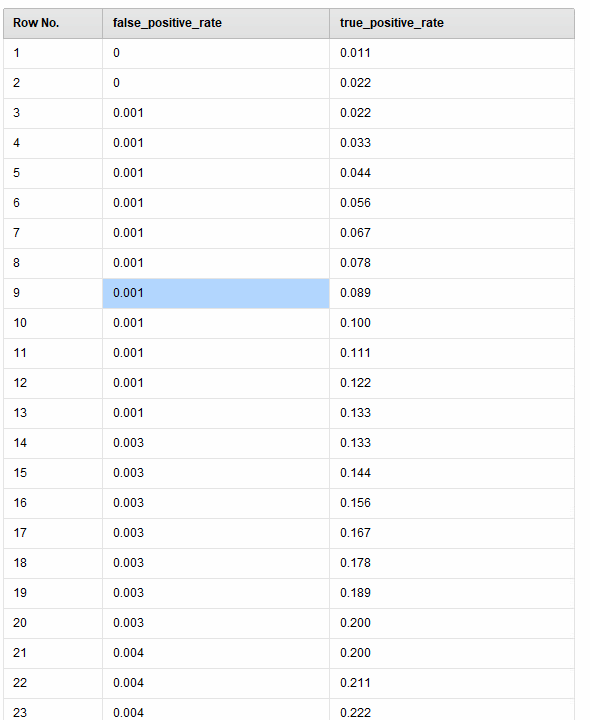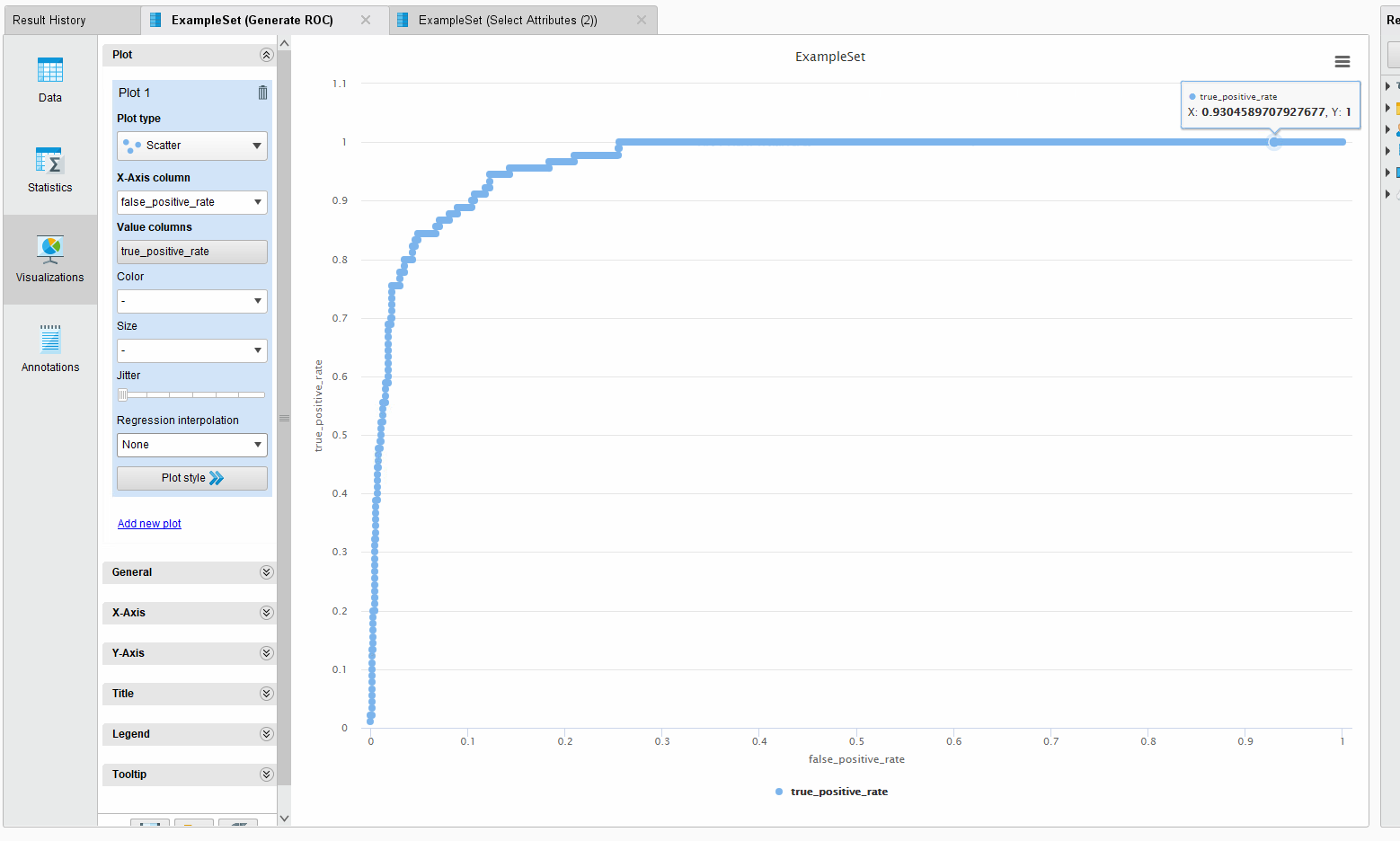# Are there any operatores that can help me draw ROC curve?

Member Posts: 13Contributor I
edited May 2020 in Help
I only get many pairs of true_positive_rate value and false positive rate value as following,I want to get ROC curve and AUC. Can your extension implement these two functions. The dataset and the process XML are in file.zip.
Tagged:

• Member Posts: 13Contributor I
I can get the ROC curve in the Visualizations of RapidMiner. Can I get the AUC from Visualizations of RapidMiner.
The picture of the result in the Visualizations of RapidMiner  is as following.• Moderator, RapidMiner Certified Analyst, Member Posts: 1,195Unicorn
Hi @liming,

If you want absolutely calculate the AUC from the FPR and TPR, here a process which performs this task :
<?xml version="1.0" encoding="UTF-8"?><process version="9.2.001">
<context>
<input/>
<output/>
<macros/>
</context>
<operator activated="true" class="process" compatibility="9.2.001" expanded="true" name="Process">
<parameter key="logverbosity" value="init"/>
<parameter key="random_seed" value="2001"/>
<parameter key="send_mail" value="never"/>
<parameter key="process_duration_for_mail" value="30"/>
<parameter key="encoding" value="SYSTEM"/>
<process expanded="true">
<parameter key="excel_file" value="C:\Users\Lionel\Documents\Formations_DataScience\Rapidminer\Tests_Rapidminer\ROC_AUC\AUC_Calculation_manually.xlsx"/>
<parameter key="sheet_selection" value="sheet number"/>
<parameter key="sheet_number" value="1"/>
<parameter key="imported_cell_range" value="A1"/>
<parameter key="encoding" value="SYSTEM"/>
<parameter key="first_row_as_names" value="true"/>
<list key="annotations"/>
<parameter key="date_format" value=""/>
<parameter key="time_zone" value="SYSTEM"/>
<parameter key="locale" value="English (United States)"/>
<list key="data_set_meta_data_information">
<parameter key="0" value="false positive rate.true.real.attribute"/>
<parameter key="1" value="true positive rate (Mean).true.real.attribute"/>
<parameter key="2" value="Aire unitaire.true.real.attribute"/>
<parameter key="3" value="Aire cumulée.true.real.attribute"/>
</list>
<parameter key="datamanagement" value="double_array"/>
<parameter key="data_management" value="auto"/>
</operator>
<operator activated="true" class="select_attributes" compatibility="9.2.001" expanded="true" height="82" name="Select Attributes" width="90" x="179" y="136">
<parameter key="attribute_filter_type" value="subset"/>
<parameter key="attribute" value=""/>
<parameter key="attributes" value="false positive rate|true positive rate (Mean)"/>
<parameter key="use_except_expression" value="false"/>
<parameter key="value_type" value="attribute_value"/>
<parameter key="use_value_type_exception" value="false"/>
<parameter key="except_value_type" value="time"/>
<parameter key="block_type" value="attribute_block"/>
<parameter key="use_block_type_exception" value="false"/>
<parameter key="except_block_type" value="value_matrix_row_start"/>
<parameter key="invert_selection" value="false"/>
<parameter key="include_special_attributes" value="false"/>
</operator>
<operator activated="true" class="time_series:lag_series" compatibility="9.2.001" expanded="true" height="82" name="Lag" width="90" x="313" y="136">
<list key="attributes">
<parameter key="false positive rate" value="1"/>
<parameter key="true positive rate (Mean)" value="1"/>
</list>
<parameter key="overwrite_attributes" value="false"/>
<parameter key="extend_exampleset" value="false"/>
</operator>
<operator activated="true" class="generate_attributes" compatibility="9.2.001" expanded="true" height="82" name="Generate Attributes" width="90" x="447" y="136">
<list key="function_descriptions">
<parameter key="AUC" value="0.5*([false positive rate]-[false positive rate-1])*([true positive rate (Mean)]+[true positive rate (Mean)-1])"/>
</list>
<parameter key="keep_all" value="true"/>
</operator>
<operator activated="true" class="aggregate" compatibility="9.2.001" expanded="true" height="82" name="Aggregate" width="90" x="581" y="136">
<parameter key="use_default_aggregation" value="false"/>
<parameter key="attribute_filter_type" value="all"/>
<parameter key="attribute" value=""/>
<parameter key="attributes" value=""/>
<parameter key="use_except_expression" value="false"/>
<parameter key="value_type" value="attribute_value"/>
<parameter key="use_value_type_exception" value="false"/>
<parameter key="except_value_type" value="time"/>
<parameter key="block_type" value="attribute_block"/>
<parameter key="use_block_type_exception" value="false"/>
<parameter key="except_block_type" value="value_matrix_row_start"/>
<parameter key="invert_selection" value="false"/>
<parameter key="include_special_attributes" value="false"/>
<parameter key="default_aggregation_function" value="average"/>
<list key="aggregation_attributes">
<parameter key="AUC" value="sum"/>
</list>
<parameter key="group_by_attributes" value=""/>
<parameter key="count_all_combinations" value="false"/>
<parameter key="only_distinct" value="false"/>
<parameter key="ignore_missings" value="true"/>
</operator>
<connect from_op="Read Excel" from_port="output" to_op="Select Attributes" to_port="example set input"/>
<connect from_op="Select Attributes" from_port="example set output" to_op="Lag" to_port="example set input"/>
<connect from_op="Lag" from_port="example set output" to_op="Generate Attributes" to_port="example set input"/>
<connect from_op="Generate Attributes" from_port="example set output" to_op="Aggregate" to_port="example set input"/>
<connect from_op="Aggregate" from_port="example set output" to_port="result 1"/>
<portSpacing port="source_input 1" spacing="0"/>
<portSpacing port="sink_result 1" spacing="0"/>
<portSpacing port="sink_result 2" spacing="0"/>
</process>
</operator>
</process>

Hope this helps,

Regards,

Lionel
• Moderator, RapidMiner Certified Analyst, Member Posts: 1,195Unicorn
Hi ,

It seems there is a displaying bug when we click on "show" on my previous post...
So here the process :

```<?xml version="1.0" encoding="UTF-8"?><process version="9.2.001">
<context>
<input/>
<output/>
<macros/>
</context>
<operator activated="true" class="process" compatibility="9.2.001" expanded="true" name="Process">
<parameter key="logverbosity" value="init"/>
<parameter key="random_seed" value="2001"/>
<parameter key="send_mail" value="never"/>
<parameter key="process_duration_for_mail" value="30"/>
<parameter key="encoding" value="SYSTEM"/>
<process expanded="true">
<parameter key="excel_file" value="C:\Users\Lionel\Documents\Formations_DataScience\Rapidminer\Tests_Rapidminer\ROC_AUC\AUC_Calculation_manually.xlsx"/>
<parameter key="sheet_selection" value="sheet number"/>
<parameter key="sheet_number" value="1"/>
<parameter key="imported_cell_range" value="A1"/>
<parameter key="encoding" value="SYSTEM"/>
<parameter key="first_row_as_names" value="true"/>
<list key="annotations"/>
<parameter key="date_format" value=""/>
<parameter key="time_zone" value="SYSTEM"/>
<parameter key="locale" value="English (United States)"/>
<list key="data_set_meta_data_information">
<parameter key="0" value="false positive rate.true.real.attribute"/>
<parameter key="1" value="true positive rate (Mean).true.real.attribute"/>
<parameter key="2" value="Aire unitaire.true.real.attribute"/>
<parameter key="3" value="Aire cumulée.true.real.attribute"/>
</list>
<parameter key="datamanagement" value="double_array"/>
<parameter key="data_management" value="auto"/>
</operator>
<operator activated="true" class="select_attributes" compatibility="9.2.001" expanded="true" height="82" name="Select Attributes" width="90" x="179" y="136">
<parameter key="attribute_filter_type" value="subset"/>
<parameter key="attribute" value=""/>
<parameter key="attributes" value="false positive rate|true positive rate (Mean)"/>
<parameter key="use_except_expression" value="false"/>
<parameter key="value_type" value="attribute_value"/>
<parameter key="use_value_type_exception" value="false"/>
<parameter key="except_value_type" value="time"/>
<parameter key="block_type" value="attribute_block"/>
<parameter key="use_block_type_exception" value="false"/>
<parameter key="except_block_type" value="value_matrix_row_start"/>
<parameter key="invert_selection" value="false"/>
<parameter key="include_special_attributes" value="false"/>
</operator>
<operator activated="true" class="time_series:lag_series" compatibility="9.2.001" expanded="true" height="82" name="Lag" width="90" x="313" y="136">
<list key="attributes">
<parameter key="false positive rate" value="1"/>
<parameter key="true positive rate (Mean)" value="1"/>
</list>
<parameter key="overwrite_attributes" value="false"/>
<parameter key="extend_exampleset" value="false"/>
</operator>
<operator activated="true" class="generate_attributes" compatibility="9.2.001" expanded="true" height="82" name="Generate Attributes" width="90" x="447" y="136">
<list key="function_descriptions">
<parameter key="AUC" value="0.5*([false positive rate]-[false positive rate-1])*([true positive rate (Mean)]+[true positive rate (Mean)-1])"/>
</list>
<parameter key="keep_all" value="true"/>
</operator>
<operator activated="true" class="aggregate" compatibility="9.2.001" expanded="true" height="82" name="Aggregate" width="90" x="581" y="136">
<parameter key="use_default_aggregation" value="false"/>
<parameter key="attribute_filter_type" value="all"/>
<parameter key="attribute" value=""/>
<parameter key="attributes" value=""/>
<parameter key="use_except_expression" value="false"/>
<parameter key="value_type" value="attribute_value"/>
<parameter key="use_value_type_exception" value="false"/>
<parameter key="except_value_type" value="time"/>
<parameter key="block_type" value="attribute_block"/>
<parameter key="use_block_type_exception" value="false"/>
<parameter key="except_block_type" value="value_matrix_row_start"/>
<parameter key="invert_selection" value="false"/>
<parameter key="include_special_attributes" value="false"/>
<parameter key="default_aggregation_function" value="average"/>
<list key="aggregation_attributes">
<parameter key="AUC" value="sum"/>
</list>
<parameter key="group_by_attributes" value=""/>
<parameter key="count_all_combinations" value="false"/>
<parameter key="only_distinct" value="false"/>
<parameter key="ignore_missings" value="true"/>
</operator>
<connect from_op="Read Excel" from_port="output" to_op="Select Attributes" to_port="example set input"/>
<connect from_op="Select Attributes" from_port="example set output" to_op="Lag" to_port="example set input"/>
<connect from_op="Lag" from_port="example set output" to_op="Generate Attributes" to_port="example set input"/>
<connect from_op="Generate Attributes" from_port="example set output" to_op="Aggregate" to_port="example set input"/>
<connect from_op="Aggregate" from_port="example set output" to_port="result 1"/>
<portSpacing port="source_input 1" spacing="0"/>
<portSpacing port="sink_result 1" spacing="0"/>
<portSpacing port="sink_result 2" spacing="0"/>
</process>
</operator>
</process>
```
Regards,

Lionel

NB : Thanks to @varunm1 to raise this error...